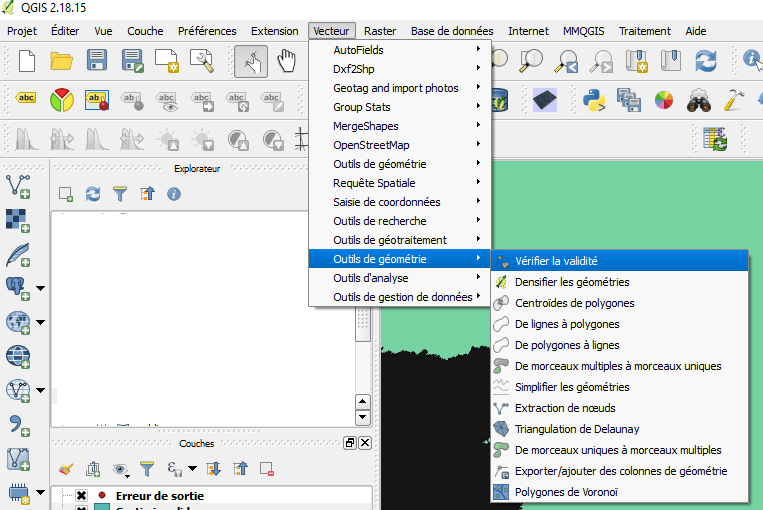# How to rectify the geometry of a Postgis table

We have already discussed the topic of geometry validation through a series of articles (in french):

In this article we will discuss, in detail, how to detect and rectify geometric problems in a PostgreSQL / Postgis table using SQL queries.

## Error checking with QGis.

You can promptly detect geometric errors using QGis. Just load the layer and launch the menu Vector – > Geometry tools – > Check the validityYou can choose one of the following  methods:

• GEOS  validation method
• QGIS validation method, and
• method  for interactive verification when creating a new geometry (Preferences → Scan tab).

The GEOS method is faster but only indicates the first error encountered for each object. It returns a file ‘Invalid output’ which is a layer of polygons completed by a column a column _errors:The Qgis method can return multiple errors per object. This method returns in the ‘Invalid output’ file a layer of polygons completed by a _errors column with a less standardized message than by the GEOS method:Personally, I only use this tool to check for errors. I execute both methods and, if they do not return any error I consider that the layer as valid.

Meanwhile,  if there are errors, I go directly to pgAdmin 4 (or failing in the database manager of QGis) to work in SQL.

## Detection of Geometric Errors with Postgis

The first step is the identification of errors. Note that PostGIS complies with the OGC OpenGIS Specifications, and therefore the validity is checked against GEOS.
Therefore, we will perform a query that will search the three main types of errors:

• GEOS errors ( see simple and valid geometries )
• Null geometries, which do not make sense
• Collections of geometries, ie objects composed by several geometries of the same type or not. The most frequent examples are multipolygons that are inaccurately cataloged as FeatureCollection . In general, spatial operators do not work with input collections.

A last type of error, usually of no consequence, is the presence of duplicated nodes (two points of the entity located exactly in the same place). They do not disturb space operators, but that’s no reason not to fix them.

Here is a query that allows you to detect the first three types of errors in one pass:

SELECT ‘non valide’ AS nb, count(*) FROM table WHERE NOT ST_IsValid(the_geom)
UNION
SELECT ‘geom nulle’ AS nb, count(*) FROM table WHERE the_geom is null
UNION
SELECT ‘collection’ AS nb, count(*) FROM table WHERE not ST_IsValid(the_geom)
AND ST_GeometryType(ST_MakeValid(the_geom))=’ST_GeometryCollection’;You can obtain details of GEOS errors with the following query:

SELECT id, ST_IsValidReason(the_geom) FROM table WHERE NOT ST_IsValid(the_geom);## Correcting errors

(We will be using  the case of a polygon layer, which is the type of entity that originates most problems.)
Correct GEOS errors with St_MakeValid

UPDATE table SET the_geom = ST_MakeValid(the_geom) WHERE NOT ST_IsValid(the_geom);

Then, we rectify  the collections by transforming them into Multi_polygons. Be careful not to reverse the steps. The St_MakeValid function can produce collections as an output.

UPDATE table
SET the_geom =
ST_Multi(ST_Simplify(ST_Multi(ST_CollectionExtract(ST_ForceCollection(ST_MakeValid(the_geom)),3)),0))
WHERE ST_GeometryType(the_geom) = ‘ST_GeometryCollection’;

Third, delete null geometries (if the error detection query found them).

DELETE FROM table WHERE the_geom IS NULL;

And finally we remove the duplicate nodes :

UPDATE table SET the_geom = ST_Multi(ST_Simplify(the_geom,0));

This last request is a, somewhat, diverted way of doing the operation. The St_Simplify function is a generalization function, ie a function that reduces the number of points of an entity, by removing points that are at a distance smaller than the parameter of the function. By setting 0 to this distance, the function leaves only one point among all points in a zero radius (duplicates).

Once these queries have been executed, the fault finding request is executed again. If errors persist, it will be necessary to use another method to solve them.

A technique often used is the creation of a null buffer:

UPDATE table SET the_geom = ST_Multi(ST_Buffer(the_geom,0)) WHERE NOT ST_IsValid(the_geom));

At this point, if errors persist, we must give up the idea of automatically correcting them, take a breath, and do it manually.

Tagged , , , , . Bookmark the permalink.

### One Response to How to rectify the geometry of a Postgis table

1.compras i ventas says:

Good post! Thanks!!